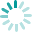## Nemilia

Baby Blanket of Trellis design, with eyelets for ribbon. http://www.ravelry.com/projects/CanadianKnitizen/nemilia

Shown with a cast-on count of 77 stitches.• Rows 1, 3, and 5 (RS): [K1, p1] 38 times, k1.
• Rows 2, 4, and 6: [K1, p1] 38 times, k1.
• Row 7: [K1, p1] twice, [yo, k2tog] 34 times, [k1, p1] twice, k1.
• Row 8: [K1, p1] twice, p1, yo, p67, [k1, p1] twice, k1 (78 sts).
• Rows 9 and 25: [K1, p1] twice, k1, yo, k1, [p1, k15] 4 times, p1, k2tog, [k1, p1] twice, k1.
• Rows 10 and 24: [K1, p1] twice, k1, yo, p2, [k1, p13, k1, p1] 4 times, p2tog, [k1, p1] twice, k1.
• Rows 11 and 23: [K1, p1] twice, k1, yo, k1, [k2, p1, k11, p1, k1] 4 times, k1, k2tog, [k1, p1] twice, k1.
• Rows 12 and 22: [K1, p1] twice, k1, yo, p1, [p3, k1, p9, k1, p2] 4 times, p1, p2tog, [k1, p1] twice, k1.
• Rows 13 and 21: [K1, p1] twice, k1, yo, k1, [k4, p1, k7, p1, k3] 4 times, k1, k2tog, [k1, p1] twice, k1.
• Rows 14 and 20: [K1, p1] twice, k1, yo, p1, [p5, k1, p5, k1, p4] 4 times, p1, p2tog, [k1, p1] twice, k1.
• Rows 15 and 19: [K1, p1] twice, k1, yo, k1, [k6, p1, k3, p1, k5] 4 times, k1, k2tog, [k1, p1] twice, k1.
• Rows 16 and 18: [K1, p1] twice, k1, yo, p1, [p7, k1, p1, k1, p6] 4 times, p1, p2tog, [k1, p1] twice, k1.
• Row 17: [K1, p1] twice, k1, yo, k1, [k8, p1, k7] 4 times, k1, k2tog, [k1, p1] twice, k1.
• Repeat rows 10-25.